## Airfoil Creation Using Inverse DesignInverse design is the process by which modern airfoils are created. A velocity profile is defined which will have the desired performance characteristics. The inverse design calculation finds the airfoil that will have the specified velocity profile.

The airfoil is divided into front segments and the rear portion that is known as the pressure recovery region.

The user begins modification of the front segments or the pressure recovery region on either the upper surface or lower surface by the option buttons. The velocity profile on the upper surface is shown in blue and on the lower surface in red.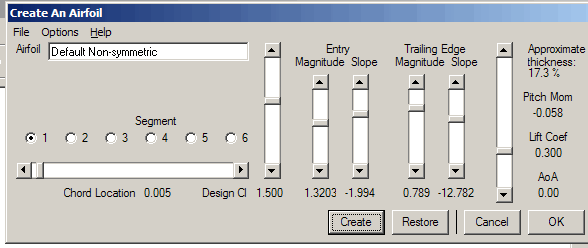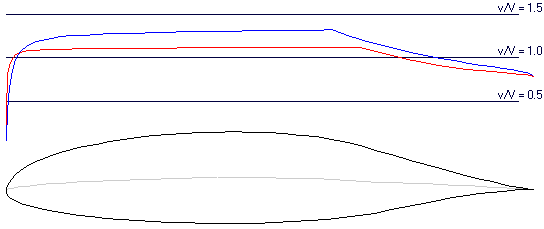As the parameters are changed, a partially converged solution to the inverse model equations is obtained. If the changes are relatively small, the partially converged solution will become more accurate as the changes are made. Each time the "Create" button is pushed, a fully converged solution is obtained. Prior to ending the creation portion of AeroFoil, the user should press the "Create" button one last time. This will ensure that fully converged airfoil is used in subsequent analysis.

In certain cases, an invalid result can be achieved. Generally, this would appear to be an airfoil that has a figure "8" shape, where the lower surface at the trailing edge is above the upper surface. When this happens, AeroFoil will display a warning message and attempt to restore the parameters back to the last valid airfoil.

Two default airfoils and several examples are available. It is easier to begin the design process using an airfoil that is similar to the desired result. Prior to ending the design process, the airfoil should be named and the parameters saved.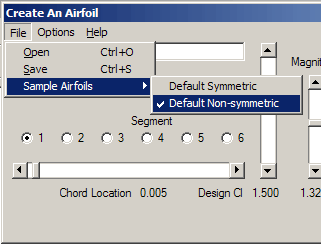## Inverse Design Mathematics

Inverse design uses a mathematical method that is similar to Joukowski conformal mapping, except that the velocity distribution is specified on the surface of the conformal mapping circle. The user defines the desired velocity profile and AeroFoil calculates the airfoil that will produce it.

Three equations must be solved in order to arrive at a mathematically valid velocity profile. After a parameter is changed, the remaining parameters are automatically adjusted until all three equations are satisfied.

Two of the three equations are satisfied by iteration on the relative magnitude of the velocity profile and the difference between the magnitude of the velocities on the upper and lower surface.

The third constraint equation is satisfied by iterating on either the "curvature" of the leading edge or the relative magnitude of the trailing edge velocity. This is a user-selected option.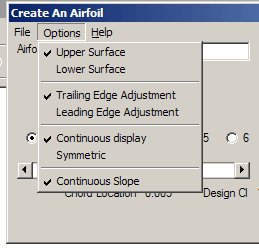## Front Segments

The front surface is divided into six different segments on both the upper and lower surfaces. The locations of the segment endpoints are shown by the large circles and may be adjusted by the horizontal slider bar. The front of the first segment begins at the leading edge of the airfoil and the front of each subsequent segment begins at the rear of the preceding segment. The horizontal slider bar will adjust the rear location of the selected segment.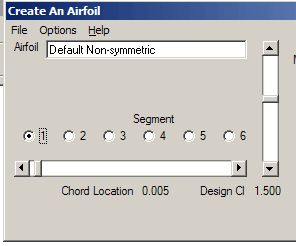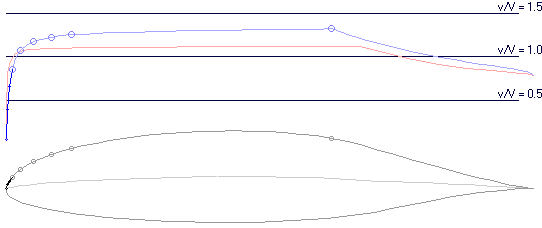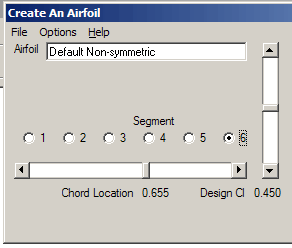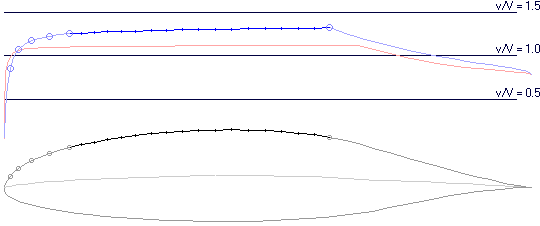The design value for the coefficient of lift for each segment represents an approximation of the coefficient of lift at which the surface velocity will be constant. These values are adjusted by the vertical slider bar.

On the upper surface at any coefficient of lift less than this design value, the surface velocity will be increasing. As the coefficient of lift is increased above the design value, surface velocity will begin to decrease. At this point, the attached boundary layer will begin to thicken and transition to turbulent or separated flow will occur. Conversely, on a bottom surface segment at any coefficient of lift greater than its design value, the surface velocity on the segment will be increasing.

The "Design Cl" is only an approximation. In a theoretical airfoil, the slope of the lift curve as a function of angle of attack is constant. In theory, the coefficient of lift will increase 0.10966 as the angle of attack increases 1 degree. In real airfoils, the slope is affected by the shape of the camber line.

In general, the magnitude of the design lift coefficient for each segment should progress from a maximum at the leading edge and decrease toward the trailing edge. This is the default and is signified by the "Continuous Slope" option selection. In this case, AeroFoil will adjust the design lift coefficients of segments both upstream and downstream of the segment that is being modified. If the "Continuous Slope" option is not selected the upstream and downstream adjustment is not made.The "Create" button will cause the calculations to be run several times until a final, fully converged, result is obtained. The "Restore" button will return the velocity profile to the most recent fully converged case.

Discontinuities in the velocity profile can sometimes be seen in the region of the leading edge and may be removed by selective adjustment of the design coefficient of lift on either the upper or the lower surface. In general, at a high angle of attack, the lower surface leading edge should be adjusted and at a negative angle of attack, the upper surface leading edge should be adjusted.

The right-most vertical slider bar alters the angle of attack. The velocity profile is re-drawn and estimated lift coefficient and pitching moment are re-calculated and displayed automatically.

## Pressure Recovery Region

The rear portion of the airfoil is an area known as the pressure recovery region. Surface velocity must decrease to some value less than the free stream velocity. The beginning of the pressure recovery region is the end of the final front segment.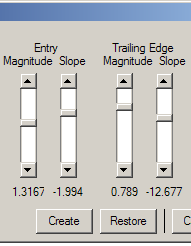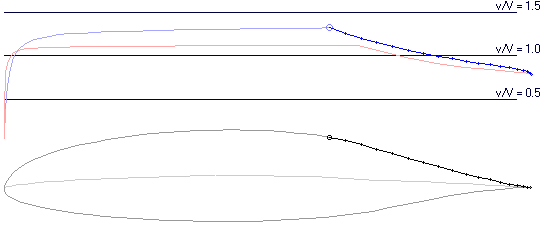The magnitude and slope of the beginning of the pressure recovery region are controlled by the first two slider bars. The magnitude and slope of the trailing edge are controlled by the second two slider bars. The relative magnitudes of velocity are shown in units of dimensional-less velocity - v/V. The magnitude of the slope is shown in units of dimensional-less velocity divided by dimensional-less length - v/V divided by x/c.

Within certain constraints, the magnitude of the trailing edge velocity may be adjusted.

If the "Trailing Edge Adjustment" option is selected and the magnitude of the trailing edge velocity is altered, then the slope of the trailing edge velocity on the selected surface is adjusted. If this adjustment continues beyond the limits set on the slope of the trailing edge velocity, then the leading edge design coefficient of lift is altered to satisfy the constraint equations.The "Create" button will cause the calculations to be run several times until a final, fully converged, result is obtained. The "Restore" button will return the velocity profile to the most recent fully converged case.

The right-most vertical slider bar alters the angle of attack. The velocity profile is re-drawn and estimated lift coefficient and pitching moment are re-calculated and displayed automatically.

## OptionsThe default selection is "trailing edge adjustment". With this option, the third constraint equation is satisfied by iterating on the relative magnitude of the trailing edge velocity. This option generally gives the most reliable results. If the "trailing edge adjustment" option is selected and the magnitude of the trailing edge velocity is altered, then the slope of the trailing edge velocity on the selected surface is adjusted. If this adjustment continues beyond the limits set on the slope of the trailing edge velocity, then the leading edge design lift coefficient is altered to satisfy the convergence.

If the "leading edge adjustment" option is selected, the third constraint equation is satisfied by iterating on the design lift coefficient at the leading edge.

If the "continuous display" option is selected, a new airfoil is displayed after every change in the velocity parameters. This is satisfactory on fast computers but it can be disabled on slower machines. In this option, the velocity profile is continuously re-calculated but the resulting airfoil is not. The calculated airfoil may be seen by depressing the "Create" button.

When selecting the symmetric case, the velocity parameters for the lower surface are set equal and opposite to those of the upper surface.

In general, the magnitude of the design lift coefficient for each segment should progress from a maximum at the leading edge and decrease toward the trailing edge. This is the default and is signified by the "continuous slope" option selection. In this case, AeroFoil will adjust the design lift coefficients of segments both upstream and downstream of the segment that is being modified. If the "continuous slope" option is not selected the upstream and downstream adjustment is not made.

The "Restore" button will return all parameters to those in effect at the last time the "Create" button was pressed.